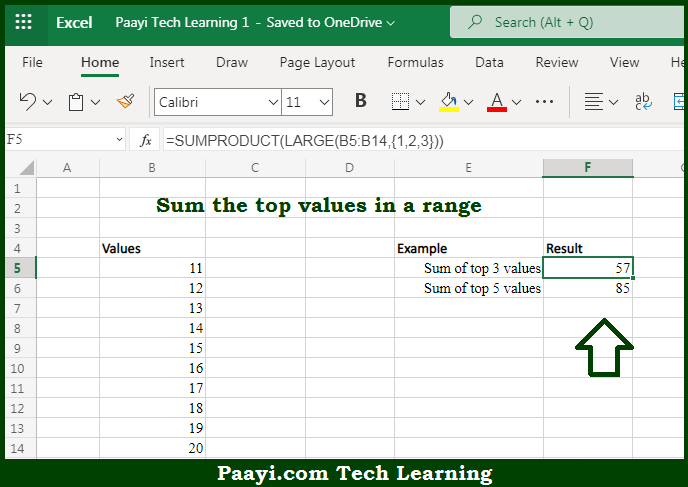# Learn How to SUM Top n Values in Microsoft Excel

Written by | 0 Comments | 580 Views

In this article, you will learn how to SUM various things in Microsoft Excel using a single/combination(s) of functions. You will also know how to SUM Top n Values and see the generic formula.

SUM Top n Values in Microsoft Excel

The main purpose of this formula is to sum the top values in a range. Here we will learn how to sum the top n values provided in the workbook in Microsoft Excel. That implies, with the help of formula based on the LARGE and SUMPRODUCT function you can able um the top values in a range,. So, with the help of this formula, you can able to sum the top n values provided in the workbook in Microsoft Excel.

General Formula to SUM Top n Values

=SUMPRODUCT(LARGE(rng,{1,2,N}))

The Explanation for the SUM Top n ValuesSo we know that with the help of the given formula above you can able to sum the top values in a range. Here we will learn how to sum the top n values provided in the workbook in Microsoft Excel. As we know that the generic form of the formula provided here, rng represents a range of cells that contain numeric values and N represents the idea of Nth value. Beside that the LARGE function will return the "Nth largest" value in a range. Also we know that SUMPRODUCT is a flexible function that allows you to uses cell references for k inside the LARGE function. So, with the help of this formula, you can able to sum the top n values provided in the workbook in Microsoft Excel.